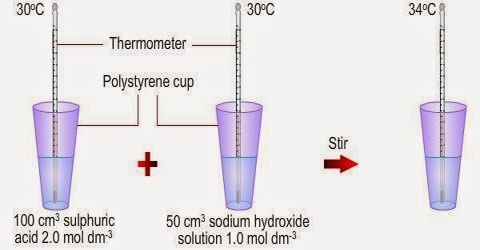# Heat of Neutralization

Heat of Neutralization

“The heat evolved when one mole of water is formed by complete neutralization of an acid with a base is called the heat of neutralization”. Heat of neutralization of strong acids by strong bases is approximately constant and is equal to about —57 Id mo1-1.The constancy of the heat of neutralization of strong acids and bases may be simply explained if one recalls that the acid-base neutralization reaction in all cases is a combination of hydrogen ions and hydroxyl ions to form undissociated water, i.e.,

H+ (aq) + OH (aq) → H2O (l)

According to the theory of electrolytes all strong acids and strong bases are completely ionized in solution. Consider the following neutralization reactions in aqueous solutions:

(a) Na+ (aq) + OH (aq) + H+ (aq) + Cl (aq) → Na+ (aq) + Cl (aq) + H2O (l)

(b) K+ (aq) + OH (aq) + H+ (aq) + NO3 (aq) → K+ (aq) + NO3 (aq) + H2O (l)

(c) Na+ (aq) + OH (aq) + H+ (aq) + ClO4 (aq) → Na+ (aq) + ClO4 (aq) + H2O (l)

In all these cases the net ionic equation far the reaction is;

H+ (aq) + OH (aq) → + H2O (l)

One mole of H+ (aq) ions react with one mole of OH (aq) ions to form one mol of H2O (l). Hence the heat of neutralization is the same, i.e., — 57 kJ mol-1 of water formed.

If, however, either the acid or the base or both are weak the heat of neutralization will not be equal to —57 kJ mol-1. This is because of incomplete dissociation of the electrolyte. For example the heat of neutralization of acetic acid by NaOH is – 55 kJ mol-1. This is because part of the heat evoked during neutralization is used up in bringing about dissociation of the weak acid (this is an endothermic process) so that one mole of water is formed. Also, since the salt formed will be that of a weak acid or base, hydrolysis will be a complicating factor. Sometimes the heat of ionization may be estimated from the observed heat of neutralization.